## Optimum Drilling Flow Rate Example Calculation

This topic will demonstrate how to determine optimum drilling flow rate for both drilling hydraulic optimization methods (the maximum hydraulic horse power and the maximum impact force). If you don’t know the equations, please read this article first, Determine Optimum Drilling Flow Rate for Basic System – Drilling Hydraulics.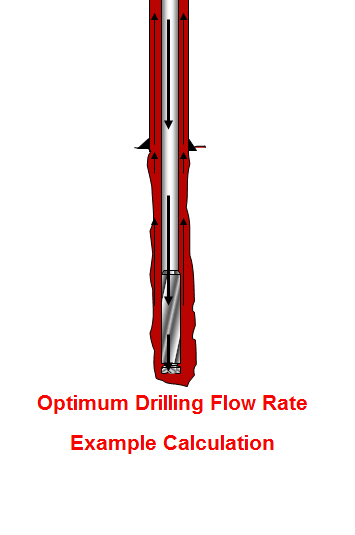The information is listed below:

Hole size = 8.5 inch

Depth In = 2,000 ft

Depth out = 6,000 ft

Mud properties at starting point

Mud weight = 9.2 ppg

PV = 13

YP = 10

## Determine Optimum Drilling Flow Rate for Basic System – Drilling Hydraulics

From the previous post (total pressure loss excluding the bit), we know the equation (shown below)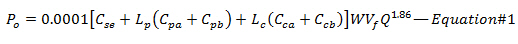We can solve the equation to get the flow rate (Q) that match with optimization criteria (Maximum Hydraulic Horsepower or Maximum Impact Force)

Maximum Hydraulic Horsepower will be happened when the pressure drop across the bit is equal to 65% of total pressure loss; therefore, 35% of total pressure loss (Ps) occurs through the drill stem (Po). The equation will be like this Po = 0.35 x Ps. By substituting Po with 0.35Ps into the Equation#1, you can solve for the optimum flow rate which will give you the maximum hydraulic horsepower.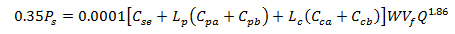Solve for Q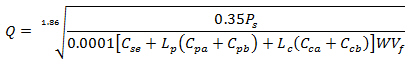Maximum Impact Force can be obtained when 48% of total pressure loss is at the bit so 52% of total pressure loss (Ps) occurs through the drill stem (Po). Substituting 52% of Ps into the Equation#1 will allow you to get the optimum flow rate at maximum impact force.Solve for Q## Total Pressure Loss through The Drilling System Without Bit

We’ve learned several calculations regarding pressure loss in the drilling system and today, we will combine all knowledge together so we will get the total pressure loss in the system excluding the bit.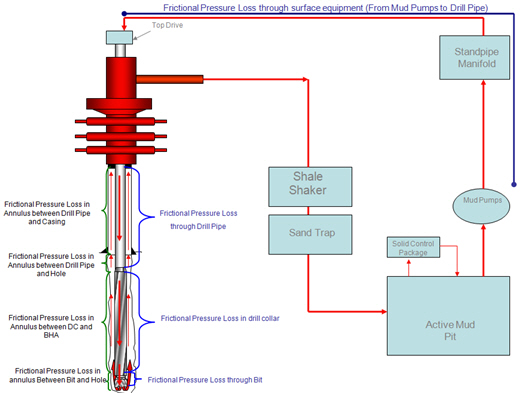Without downhole tool, the total pressure loss without bit is equal to summation of pressure loss at the surface equipment, pressure loss through drill string (drillpipe and drill collar) and pressure loss in the annulus.

The equation below demonstrates the system pressure loss excluding the bit:## Coefficient For Annulus Around Drill Pipe and Drill Collar

A term “C” represents the general coefficient for annulus around drill pipe and drill collar and the equation is as follow: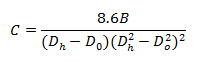Where:

Dh = hole diameter, inch

Do = Outside diameter of drill pipe or drill collar, inch

B = a parameter which takes into account the difference between Q1.86 and Q2

This term does not consider the different between size of tool joint and pipe which affect pressure drop in the annulus. This topic will demonstrate equations to get more accurate coefficient.

Coefficient of Annulus around Drill Pipe and Tool Joint

In order to get an accurate annulus pressure drop around a drill string, the coefficient for the annulus around tool joint must be taken in to account.## Pressure Losses Through Annulus

Pressure drop in the annulus is another important part of drilling hydraulic calculation and today we would like to demonstrate the equations specifically used to determine pressure loss in the annulus.The following equations are used for calculating pressure loss in the annulus.